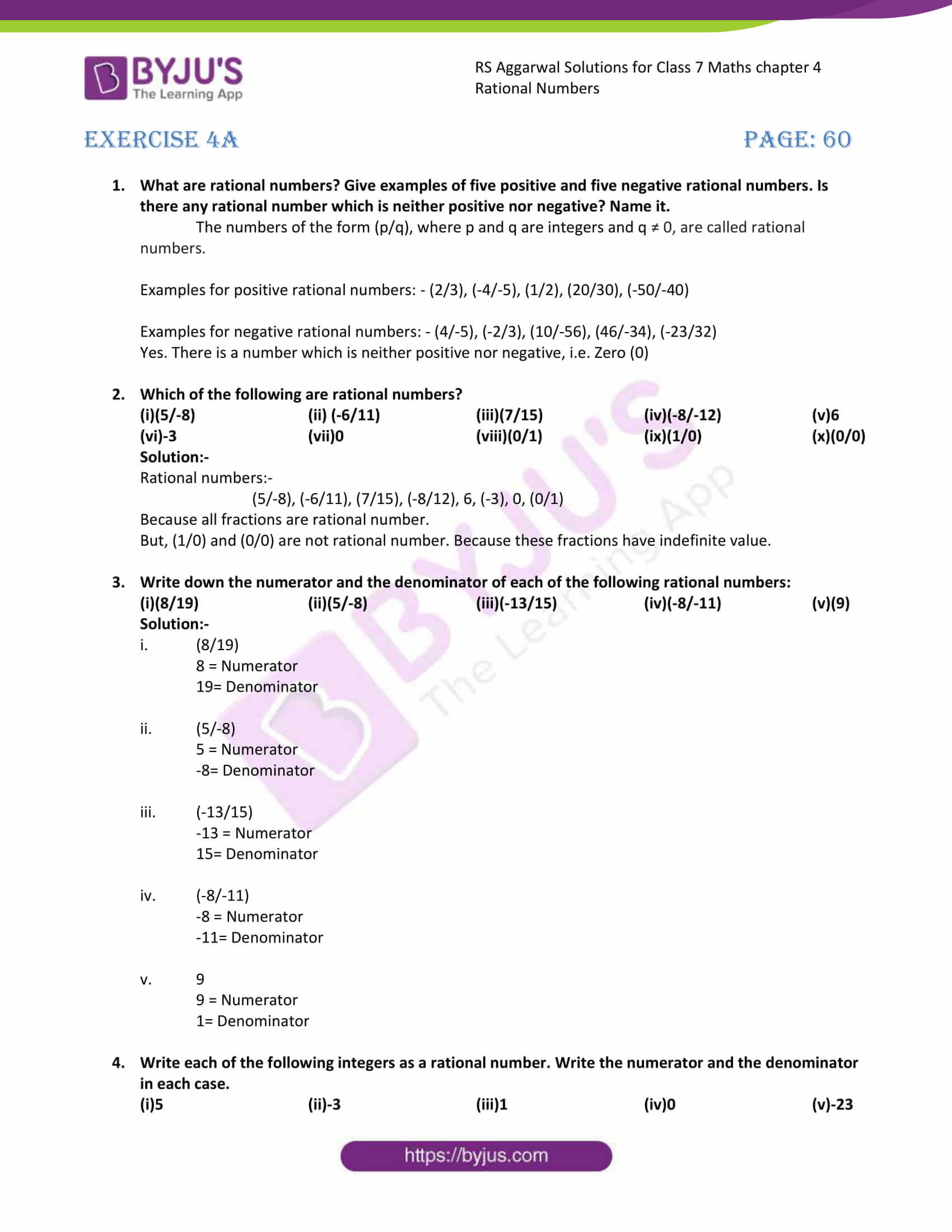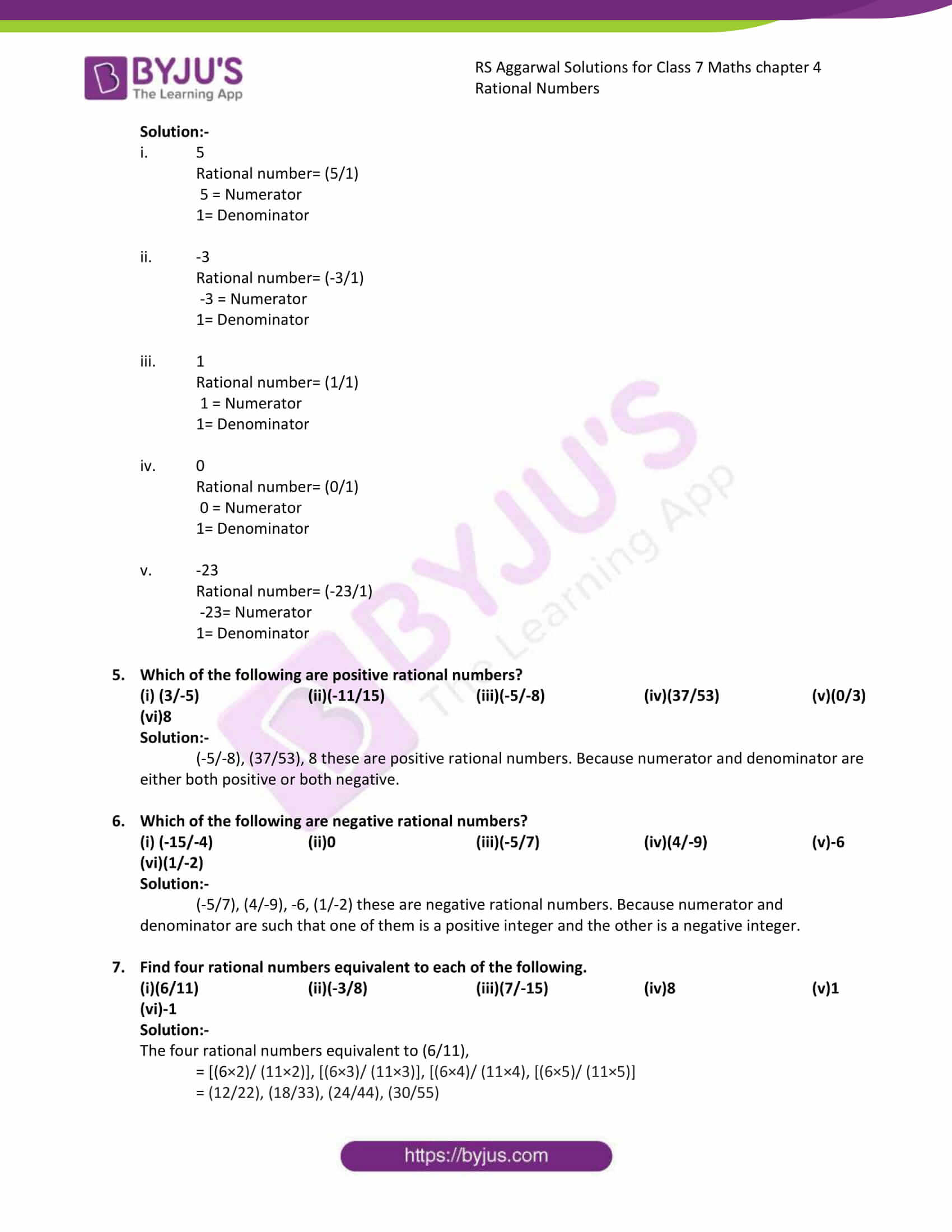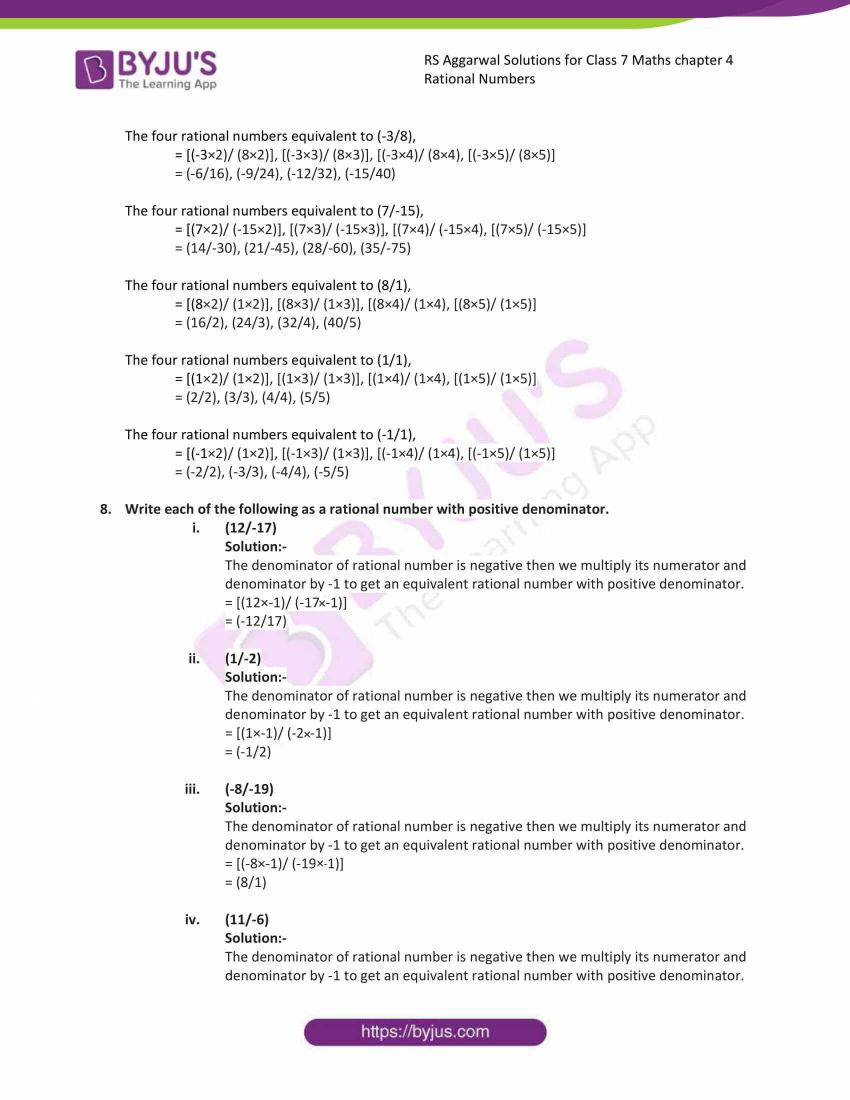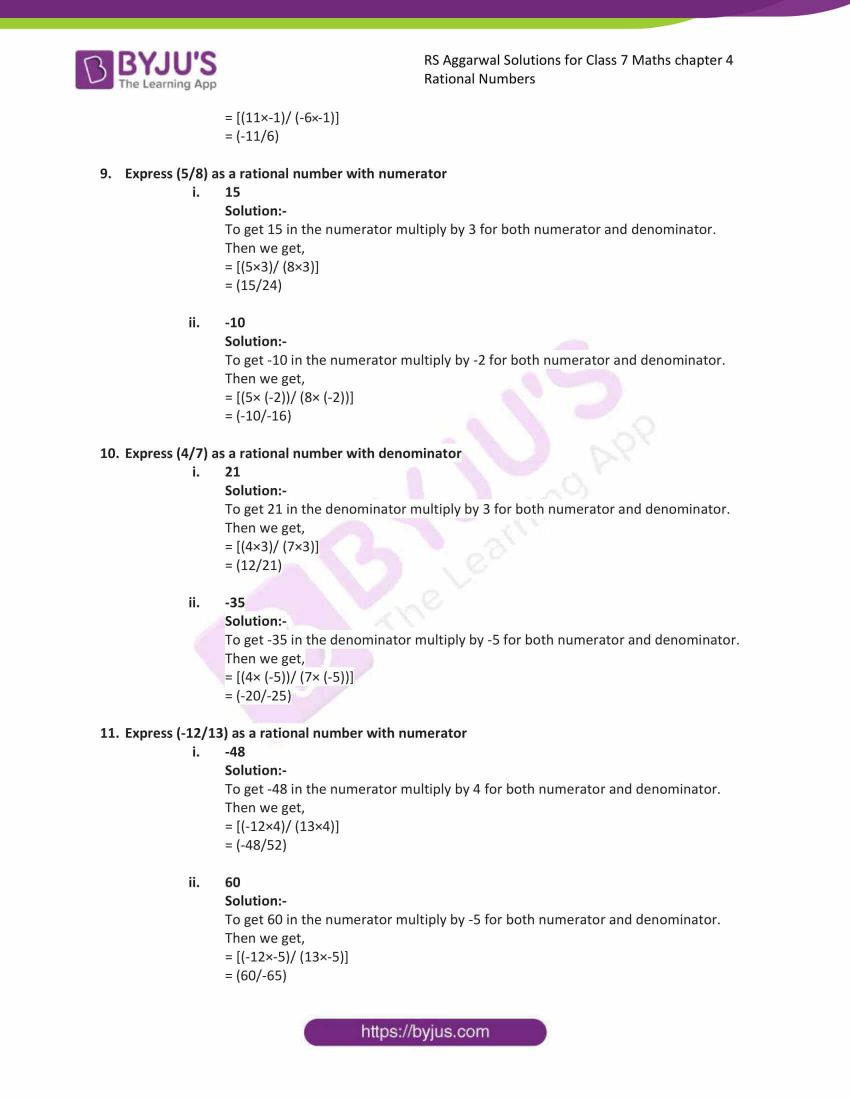# RS Aggarwal Solutions for Class 7 Maths Exercise 4A Chapter 4 Rational Numbers

RS Aggarwal Solutions for Class 7 Maths Exercise 4A Chapter 4 Rational Numbers in simple PDF are available here. This exercise of RS Aggarwal Solutions for Class 7 Maths Chapter 4 Rational Numbers has topics related to equivalent rational numbers and standard form of the rational numbers. On multiplying the numerator and denominator of a given rational number by the same nonzero number, we get a rational number equivalent to the given rational number. A rational number (p/q) is said to be in standard form, if q is positive, and p and q have no common divisor other than 1. Students are suggested to try solving the questions from RS Aggarwal Solutions for Class 7 Maths Exercise 4A Chapter 4 Rational Numbers.

## Download the PDF of RS Aggarwal Solutions For Class 7 Maths Chapter 4 Rational Numbers – Exercise 4A### Access answers to Maths RS Aggarwal Solutions for Class 7 Chapter 4 – Rational Numbers Exercise 4A

1. What are rational numbers? Give examples of five positive and five negative rational numbers. Is there any rational number which is neither positive nor negative? Name it.

The numbers of the form (p/q), where p and q are integers and q ≠ 0, are called rational numbers.

Examples for positive rational numbers: – (2/3), (-4/-5), (1/2), (20/30), (-50/-40)

Examples for negative rational numbers: – (4/-5), (-2/3), (10/-56), (46/-34), (-23/32)

Yes. There is a number which is neither positive nor negative, i.e. Zero (0)

2. Which of the following are rational numbers?

(i)(5/-8) (ii)(-6/11) (iii)(7/15) (iv)(-8/-12) (v)6 (vi)-3 (vii)0 (viii)(0/1) (ix)(1/0) (x)(0/0)

Solution:-

Rational numbers:-

(5/-8), (-6/11), (7/15), (-8/12), 6, (-3), 0, (0/1)

Because all fractions are rational number.

But, (1/0) and (0/0) are not rational number. Because these fractions have indefinite value.

3. Write down the numerator and the denominator of each of the following rational numbers:

(i)(8/19) (ii)(5/-8) (iii)(-13/15) (iv)(-8/-11) (v)(9)

Solution:-

(i) (8/19)

8 = Numerator

19= Denominator

(ii) (5/-8)

5 = Numerator

-8= Denominator

(iii) (-13/15)

-13 = Numerator

15= Denominator

(iv) (-8/-11)

-8 = Numerator

-11= Denominator

(v) 9

9 = Numerator

1= Denominator

4. Write each of the following integers as a rational number. Write the numerator and the denominator in each case.

(i)5 (ii)-3 (iii)1 (iv)0 (v)-23

Solution:-

(i) 5

Rational number= (5/1)

5 = Numerator

1= Denominator

(ii) -3

Rational number= (-3/1)

-3 = Numerator

1= Denominator

(iii) 1

Rational number= (1/1)

1 = Numerator

1= Denominator

(iv) 0

Rational number= (0/1)

0 = Numerator

1= Denominator

(v) -23

Rational number= (-23/1)

-23= Numerator

1= Denominator

5. Which of the following are positive rational numbers?

(i) (3/-5) (ii)(-11/15) (iii)(-5/-8) (iv)(37/53) (v)(0/3)

(vi)8

Solution:-

(-5/-8), (37/53), 8 these are positive rational numbers. Because numerator and denominator are either both positive or both negative.

6. Which of the following are negative rational numbers?

(i) (-15/-4) (ii)0 (iii)(-5/7) (iv)(4/-9) (v)-6

(vi)(1/-2)

Solution:-

(-5/7), (4/-9), -6, (1/-2) these are negative rational numbers. Because numerator and denominator are such that one of them is a positive integer and the other is a negative integer.

7. Find four rational numbers equivalent to each of the following.

(i)(6/11) (ii)(-3/8) (iii)(7/-15) (iv)8 (v)1

(vi)-1

Solution:-

The four rational numbers equivalent to (6/11),

= [(6×2)/ (11×2)], [(6×3)/ (11×3)], [(6×4)/ (11×4), [(6×5)/ (11×5)]

= (12/22), (18/33), (24/44), (30/55)

The four rational numbers equivalent to (-3/8),

= [(-3×2)/ (8×2)], [(-3×3)/ (8×3)], [(-3×4)/ (8×4), [(-3×5)/ (8×5)]

= (-6/16), (-9/24), (-12/32), (-15/40)

The four rational numbers equivalent to (7/-15),

= [(7×2)/ (-15×2)], [(7×3)/ (-15×3)], [(7×4)/ (-15×4), [(7×5)/ (-15×5)]

= (14/-30), (21/-45), (28/-60), (35/-75)

The four rational numbers equivalent to (8/1),

= [(8×2)/ (1×2)], [(8×3)/ (1×3)], [(8×4)/ (1×4), [(8×5)/ (1×5)]

= (16/2), (24/3), (32/4), (40/5)

The four rational numbers equivalent to (1/1),

= [(1×2)/ (1×2)], [(1×3)/ (1×3)], [(1×4)/ (1×4), [(1×5)/ (1×5)]

= (2/2), (3/3), (4/4), (5/5)

The four rational numbers equivalent to (-1/1),

= [(-1×2)/ (1×2)], [(-1×3)/ (1×3)], [(-1×4)/ (1×4), [(-1×5)/ (1×5)]

= (-2/2), (-3/3), (-4/4), (-5/5)

8. Write each of the following as a rational number with positive denominator.
(i) (12/-17)

Solution:-

The denominator of rational number is negative then we multiply its numerator and denominator by -1 to get an equivalent rational number with positive denominator.

= [(12×-1)/ (-17×-1)]

= (-12/17)

(ii) (1/-2)

Solution:-

The denominator of rational number is negative then we multiply its numerator and denominator by -1 to get an equivalent rational number with positive denominator.

= [(1×-1)/ (-2×-1)]

= (-1/2)

(iii) (-8/-19)

Solution:-

The denominator of rational number is negative then we multiply its numerator and denominator by -1 to get an equivalent rational number with positive denominator.

= [(-8×-1)/ (-19×-1)]

= (8/1)

(iv) (11/-6)

Solution:-

The denominator of rational number is negative then we multiply its numerator and denominator by -1 to get an equivalent rational number with positive denominator.

= [(11×-1)/ (-6×-1)]

= (-11/6)

9. Express (5/8) as a rational number with numerator
(i) 15

Solution:-

To get 15 in the numerator multiply by 3 for both numerator and denominator.

Then we get,

= [(5×3)/ (8×3)]

= (15/24)

(ii) -10

Solution:-

To get -10 in the numerator multiply by -2 for both numerator and denominator.

Then we get,

= [(5× (-2))/ (8× (-2))]

= (-10/-16)

10. Express (4/7) as a rational number with denominator
(i) 21

Solution:-

To get 21 in the denominator multiply by 3 for both numerator and denominator.

Then we get,

= [(4×3)/ (7×3)]

= (12/21)

(ii) -35

Solution:-

To get -35 in the denominator multiply by -5 for both numerator and denominator.

Then we get,

= [(4× (-5))/ (7× (-5))]

= (-20/-25)

11. Express (-12/13) as a rational number with numerator
(i) -48

Solution:-

To get -48 in the numerator multiply by 4 for both numerator and denominator.

Then we get,

= [(-12×4)/ (13×4)]

= (-48/52)

(ii) 60

Solution:-

To get 60 in the numerator multiply by -5 for both numerator and denominator.

Then we get,

= [(-12×-5)/ (13×-5)]

= (60/-65)

### Access other exercises of RS Aggarwal Solutions For Class 7 Maths Chapter 4 – Rational Numbers

Exercise 4B Solutions

Exercise 4C Solutions

Exercise 4D Solutions

Exercise 4E Solutions

Exercise 4F Solutions

Exercise 4G Solutions